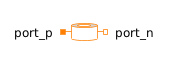Polar To Complex - MapleSim Help

Polar To Complex

Converts polar representation to complexDescription The Polar To Complex component converts real inputs in polar form to a complex output.Equations $y=\mathrm{len}\left(\mathrm{cos}\left(\mathrm{\phi }\right)+I\mathrm{sin}\left(\mathrm{\phi }\right)\right)$Connections

 Name Description Modelica ID $y$ Connector of Complex output signal y $\mathrm{len}$ Real input; length of complex signal len $\mathrm{\phi }$ Real input; angle of complex signal phiModelica Standard Library The component described in this topic is from the Modelica Standard Library. To view the original documentation, which includes author and copyright information, click here.